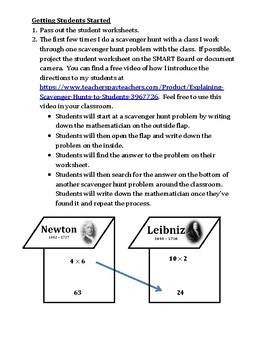# Multiplication Facts Scavenger Hunt GameSubject
Resource Type
File Type

PDF

(1 MB|37 pages)
Standards
Also included in:
1. Scavenger hunts are an excellent activity to get students moving around the classroom while being engaged with the mathematics. In these activities, students use the answer to one problem to find another problem hanging around the classroom. The student worksheets, scavenger hunt problems, instruc
\$21.00
\$18.90
Save \$2.10
• Product Description
• StandardsNEW

Do you need a fun and engaging way for students to practice multiplying with minimal prep? The Multiplication Facts Scavenger Hunt Game gets students up and moving around while practicing math. In this game, students use the answer to one problem to find another problem hanging around the classroom. Students will complete a total of 16 problems to create a list of mathematicians. The student worksheet, scavenger hunt problems, instructions, and teacher’s key are all included. These scavenger hunts are great for students who need to move around and have a hard time staying on task. It is self-checking and allows me to work one-on-one with students who need remediation or enrichment. My students love seeing scavenger hunts on the agenda.

Problems require students to:

• Know multiplication facts up to 10

This product is also included in the Multiplying Scavenger Hunt Bundle.

You may also be interested in:

Multiplication Facts Scavenger Hunt Game

Multiplying Two-Digit Numbers by One-Digit Numbers Scavenger Hunt Game

Multiplying Three-Digit Numbers by One-Digit Numbers Scavenger Hunt Game

Multiplying Four- and Five-Digit Numbers by One-Digit Numbers Scavenger Hunt Game

Multiplying Two-Digit Numbers by Two-Digit Numbers Scavenger Hunt Game

Multiplying Three-Digit Numbers by Two-Digit Numbers Scavenger Hunt Game

Multiplying Four-Digit Numbers by Two-Digit Numbers Scavenger Hunt Game

Multiplying Review Scavenger Hunt Game

Thank you to Tracee Orman for the awesome clipart.

Multiply a whole number of up to four digits by a one-digit whole number, and multiply two two-digit numbers, using strategies based on place value and the properties of operations. Illustrate and explain the calculation by using equations, rectangular arrays, and/or area models.
Multiply one-digit whole numbers by multiples of 10 in the range 10–90 (e.g., 9 × 80, 5 × 60) using strategies based on place value and properties of operations.
Total Pages
37 pages
Included
Teaching Duration
30 minutes
Report this Resource to TpT
Reported resources will be reviewed by our team. Report this resource to let us know if this resource violates TpT’s content guidelines.